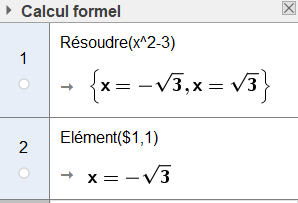# Extract solutions in Solve command in real number

Nguyễn Ngọc Tự shared this question 1 year ago

As we known, Solve Command return real numbers symbolically

ex: Solve(x^2-3) return list={x=-sqrt(3), x=sqrt(3)}

If we call list(1), it returns the round number x=-1.73.

Are there any way to extract that list in the "real number form" such as call list(1) will return x=-sqrt(3) or -sqrt(3)

Thank you very much2

je suppose que vous êtes en fenêtre CAS View, essayez Element()1

Hi Noel Lambert,

I'll try your suggestion but it does not work. Cannot edit eq bay \$eq. It works only on CAS and how to call that value to graphics?1

Thanks Noel Lambert,FormulaText(\$2) works on graphics.2